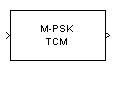# M-PSK TCM Decoder

Decode trellis-coded modulation data, modulated using PSK method

## Library

TCM, in Digital Baseband sublibrary of Modulation

•## Description

The M-PSK TCM Decoder block uses the Viterbi algorithm to decode a trellis-coded modulation (TCM) signal that was previously modulated using a PSK signal constellation.

The M-ary number parameter represents the number of points in the signal constellation, which also equals the number of possible output symbols from the convolutional encoder. (That is, log2(M-ary number) is the number of output bit streams from the convolutional encoder.)

The Trellis structure and M-ary number parameters in this block should match those in the M-PSK TCM Encoder block, to ensure proper decoding.

### Input and Output Signals

This block accepts a column vector input signal containing complex numbers. The input signal must be `double` or `single`. The reset port signal must be `double` or `Boolean`. For information about the data types each block port supports, see Supported Data Types.

If the convolutional encoder described by the trellis structure represents a rate k/n code, then the M-PSK TCM Decoder block's output is a binary column vector whose length is k times the vector length of the input signal.

### Operation Modes

The block has three possible methods for transitioning between successive frames. The Operation mode parameter controls which method the block uses. This parameter also affects the range of possible values for the Traceback depth parameter, D.

• In `Continuous` mode, the block initializes all state metrics to zero at the beginning of the simulation, waits until it accumulates D symbols, and then uses a sequence of D symbols to compute each of the traceback paths. D can be any positive integer. At the end of each frame, the block saves its internal state metric for use with the next frame.

If you select Enable the reset input, the block displays another input port, labeled `Rst`. This port receives an integer scalar signal. Whenever the value at the `Rst` port is nonzero, the block resets all state metrics to zero and sets the traceback memory to zero.

• In `Truncated` mode, the block treats each frame independently. The traceback path starts at the state with the lowest metric. D must be less than or equal to the vector length of the input.

• In `Terminated` mode, the block treats each frame independently. The traceback path always starts at the all-zeros state. D must be less than or equal to the vector length of the input. If you know that each frame of data typically ends at the all-zeros state, then this mode is an appropriate choice.

### Decoding Delay

If you set Operation mode to `Continuous`, then this block introduces a decoding delay equal to Traceback depth*k bits, for a rate k/n convolutional code. The decoding delay is the number of zeros that precede the first decoded bit in the output.

The block incurs no delay for other values of Operation mode.

## Parameters

Trellis structure

MATLAB® structure that contains the trellis description of the convolutional encoder.

M-ary number

The number of points in the signal constellation.

Traceback depth

The number of trellis branches (equivalently, the number of symbols) the block uses in the Viterbi algorithm to construct each traceback path.

Operation mode

The operation mode of the Viterbi decoder. Choices are `Continuous`, `Truncated`, and `Terminated`.

Enable the reset input port

When you check this box, the block has a second input port labeled `Rst`. Providing a nonzero input value to this port causes the block to set its internal memory to the initial state before processing the input data. This option appears only if you set Operation mode to `Continuous`.

Output data type

The output type of the block can be specified as a `boolean` or `double`. By default, the block sets this to `double`.

## Supported Data Types

PortSupported Data Types

Input

• Double-precision floating point

• Single-precision floating point

Reset

• Double-precision floating point

• Boolean

Output

• Double-precision floating point

• Boolean

## Pair Block

M-PSK TCM Encoder

## References

 Biglieri, E., D. Divsalar, P. J. McLane and M. K. Simon, Introduction to Trellis-Coded Modulation with Applications, New York, Macmillan, 1991.

 Proakis, John G., Digital Communications, Fourth edition, New York, McGraw-Hill, 2001.